# 力扣树专题-4 用leetcode104求树的深度举例 总结递归秒杀树问题的套路！ java刷题笔记

【图片源自b站 迷糊老师】hhh2021.6.25 更新 leetcode110 平衡二叉树 练一下吧朋友们 一模一样的思路

• 打开104. 二叉树的最大深度
• 打开本文
用个30-60min 把这题以及相关方法整明白
啥？时间不够？收藏下慢慢看呗[doge]

（之后我要是遇到可以用这类方法解决的题目 会更新进来的~）# 104.二叉树的最大深度

104. 二叉树的最大深度 easy

    3
/ \
9  20
/  \
15   7


## 解题思路

【1】自顶向下

21.6.26更新 这里注意 自顶向下 对于同一个节点，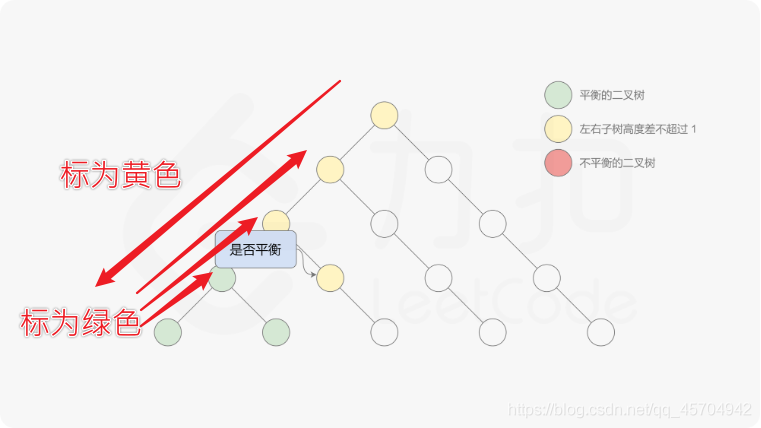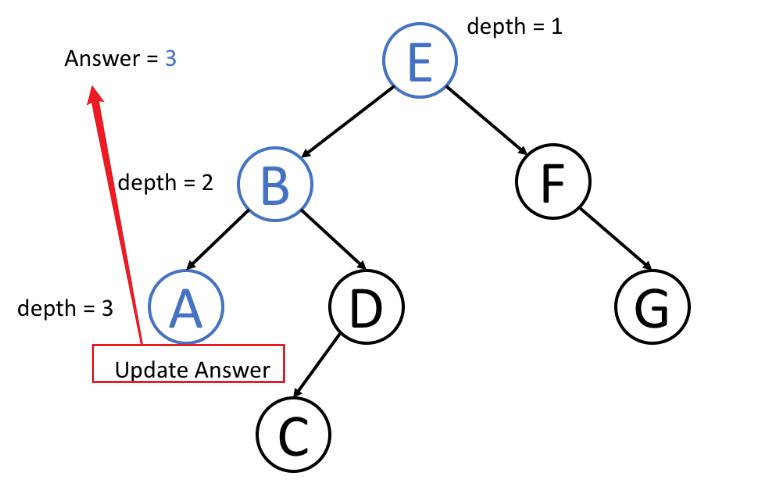【2】自底向上

21.6.26更新 这里注意 自底向上 注意这个方法是“提前阻断”的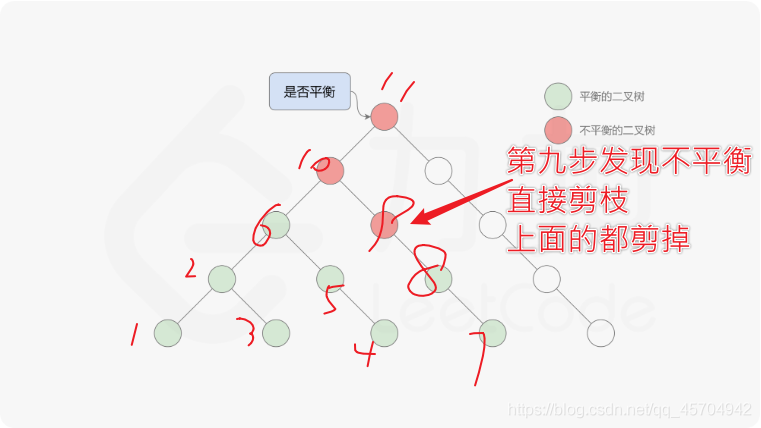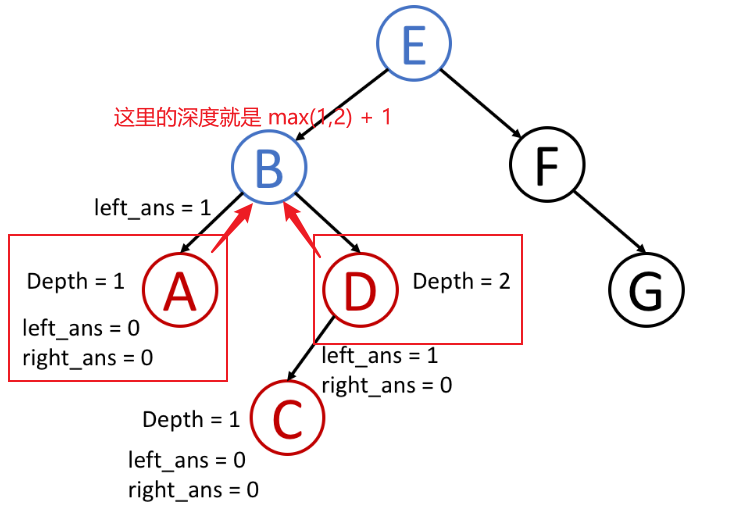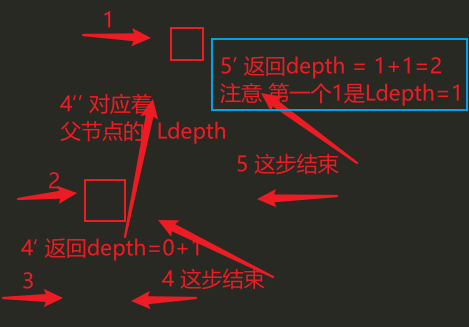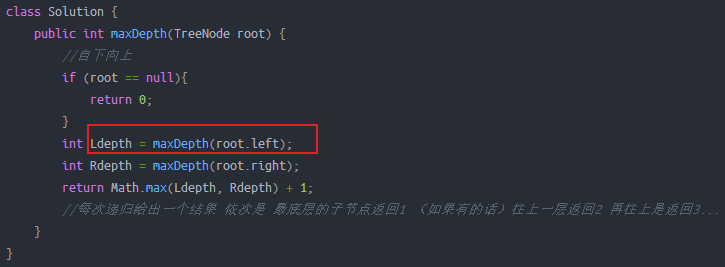## 递归 自顶向下

class Solution {
public int ans = 0;
public void _maxDepth(TreeNode root, int depth) {
if (root == null) return;
if (root.left == null && root.right == null){
ans = Math.max(ans, depth);//到最底下更新一下 ans
}
_maxDepth(root.left, depth + 1);//逐层的深入 每进入新的一层 就把深度加一~
_maxDepth(root.right, depth + 1);//左边深入完 再来深入右边~
}
public int maxDepth(TreeNode root){
_maxDepth(root, 1);
return ans;
}
}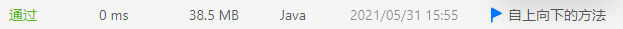## 递归 自底向上

class Solution {
public int maxDepth(TreeNode root) {
//自下向上
if (root == null){
return 0;
}
int Ldepth = maxDepth(root.left);
int Rdepth = maxDepth(root.right);
return Math.max(Ldepth, Rdepth) + 1;
//每次递归给出一个结果 依次是 最底层的子节点返回1 （如果有的话）往上一层返回2 再往上是返回3...
}
}## 利用三元表达式实现一行递归

class Solution{
public int maxDepth(TreeNode root){
return root == null ? 0 : Math.max( maxDepth(root.left), maxDepth(root.right) ) + 1;
}
}# 运用递归解决树的问题

（同样采用了leetcode104这个例子~）

## 1.自顶向下

• 访问节点来计算一些值
• 递归调用函数时将这些值传递到子节点
• 传递过程中达到一定条件就更新结果

【0】设置好最终答案 设置好递归函数 (输入节点， 输入临时答案) 函数用于计算出答案 answer

【1】设置特殊值退出条件

【2】遍历到某个节点时 更新答案

【3】遍历左子树 & 遍历右子树

return specific value for null node
left_ans = top_down(root.left, left_params)		// left_params <-- root.val, params
right_ans = top_down(root.right, right_params)	// right_params <-- root.val, params


### 求二叉树深度的例子

return if root is null
if root is a leaf node:
maximum_depth(root.left, depth + 1)      // call the function recursively for left child
maximum_depth(root.right, depth + 1)		// call the function recursively for right child




private int answer = 0;
private void maximum_depth(TreeNode root, int depth)




if (root == null){
return;
}




if (root.left == null && root.right == null){
}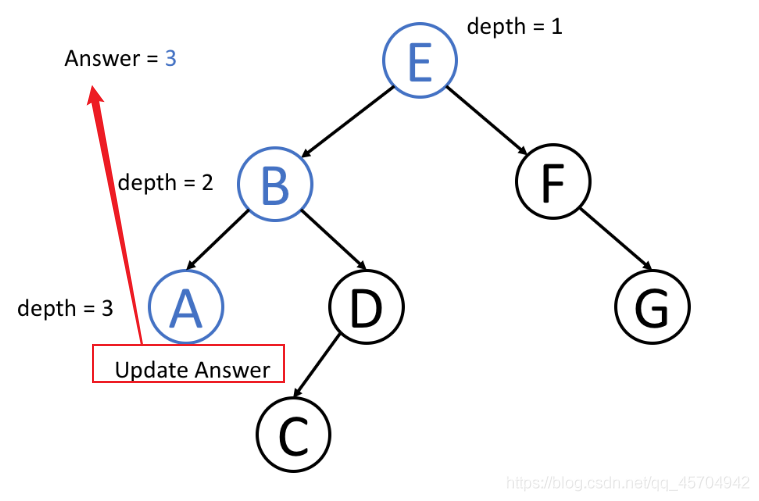maximum_depth(root.left, depth + 1);//遍历左子树 同时让深度+1
maximum_depth(root.right, depth + 1);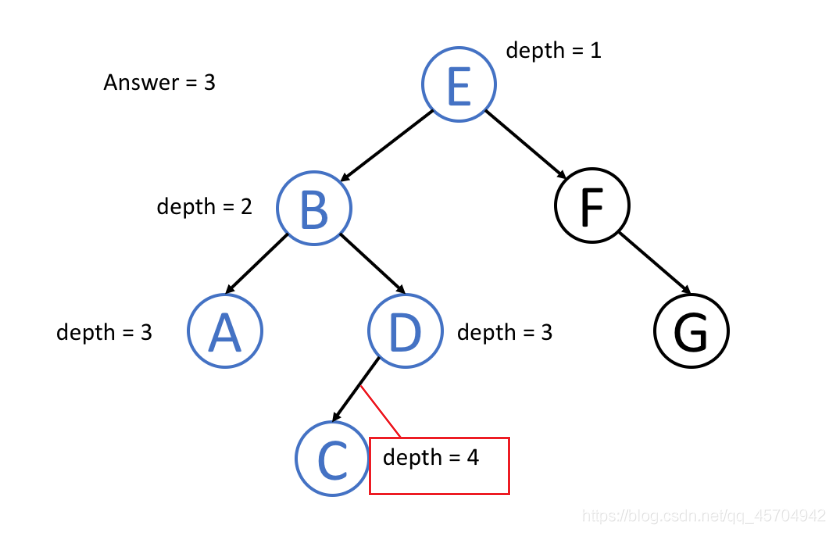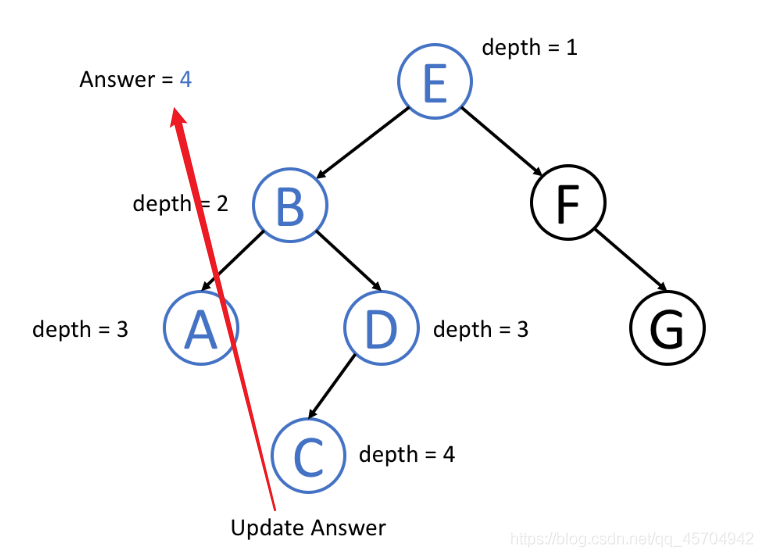## 2.自底向上

• 首先对所有子节点递归地使用调用函数
• 然后根据返回值和根节点本身的值得到答案

【0】设置好递归函数（只需要输入节点即可） 函数可以直接返回答案

【1】设置特殊值退出条件

【2】对左右子树调用递归函数

【3】左子树返回一个值 右子树返回一个值 最后将两边的值进行汇总得到最终解

return specific value for null node
left_ans = bottom_up(root.left)			// call function recursively for left child
right_ans = bottom_up(root.right)		// call function recursively for right child


### 求二叉树深度的例子

return 0 if root is null                 // return 0 for null node
left_depth = maximum_depth(root.left)
right_depth = maximum_depth(root.right)
return max(left_depth, right_depth) + 1	// return depth of the subtree rooted at root




public int maximum_depth(TreeNode root)




if (root == null){
return 0;
}




int left_depth = maximum_depth(root.left);
int right_depth = maximum_depth(root.right);




return Math.max(left_depth, right_depth) + 1;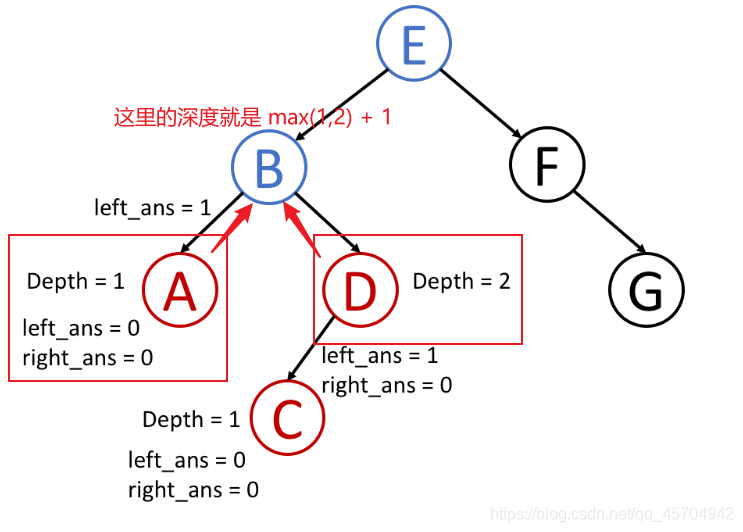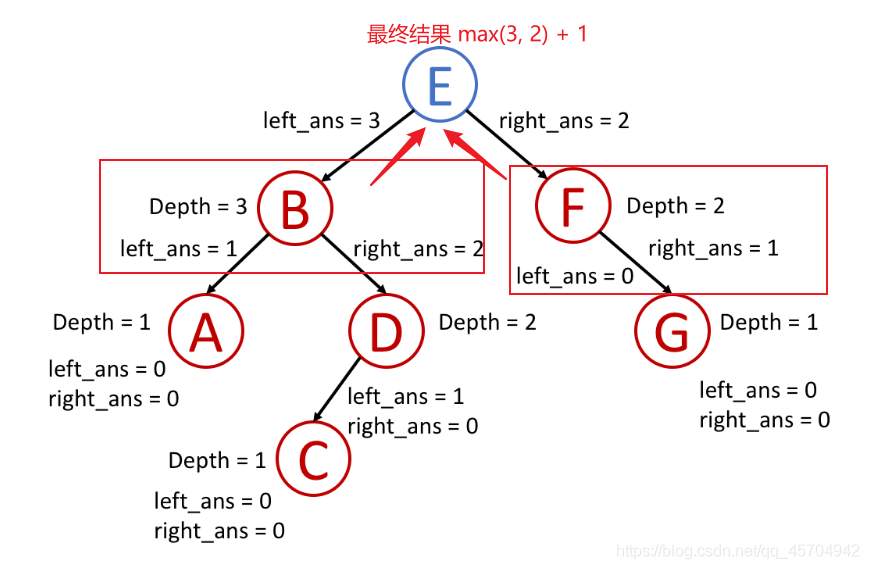## 3.递归秒杀二叉树套路！

• 是否可以确定一些参数 在每一步节点的遍历中 都能更新这些参数 最终达到答案

（可以参考上面举 出 的 自 顶 向 下 求 深 度 的 例 子 举出的自顶向下求深度的例子 就是每一步都让参数depth ++）

• 是否可以使用这些参数和节点本身的值来决定什么应该是传递给它子节点的参数

• 对于树中任意一个节点 如果你知道它子节点的答案 你能计算出该节点的答案嘛？

（参考上面自 下 向 上 求 深 度 自下向上求深度的例子 如果你知道了一个节点左右子树的深度 那这个节点的深度自然而然就是 left_ans + right_ans + 1 )

## 4.小结

• 从根开始一点点往下更新结果
• 或者先遍历到节点 一点点往上更新结果